# Numerical Solution of Resonant Layer Equations

It is possible to solve the resonant layer equation (5.78) numerically. We already know that, in the small-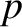limit, the solution to this equation takes the form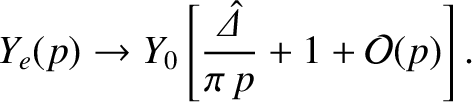(5.115)

[See Equation (5.83).] In the large-limit, Equations (5.78)–(5.81) reduce to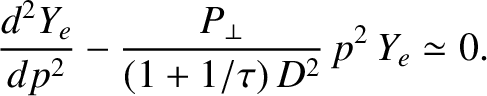(5.116)

This is a parabolic cylinder equation  whose most general large-solution is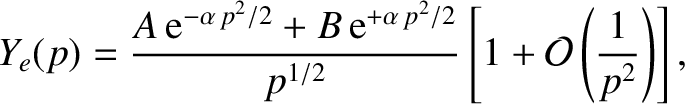(5.117)

where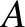and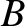are arbitrary constants, and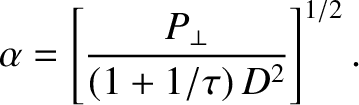(5.118)

Obviously, the physical solution of Equation (5.116) does not blow up at large. Hence, we must select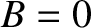in Equation (5.117), which implies that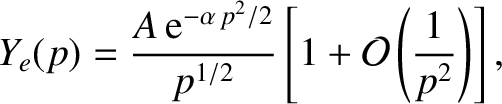(5.119)

at large.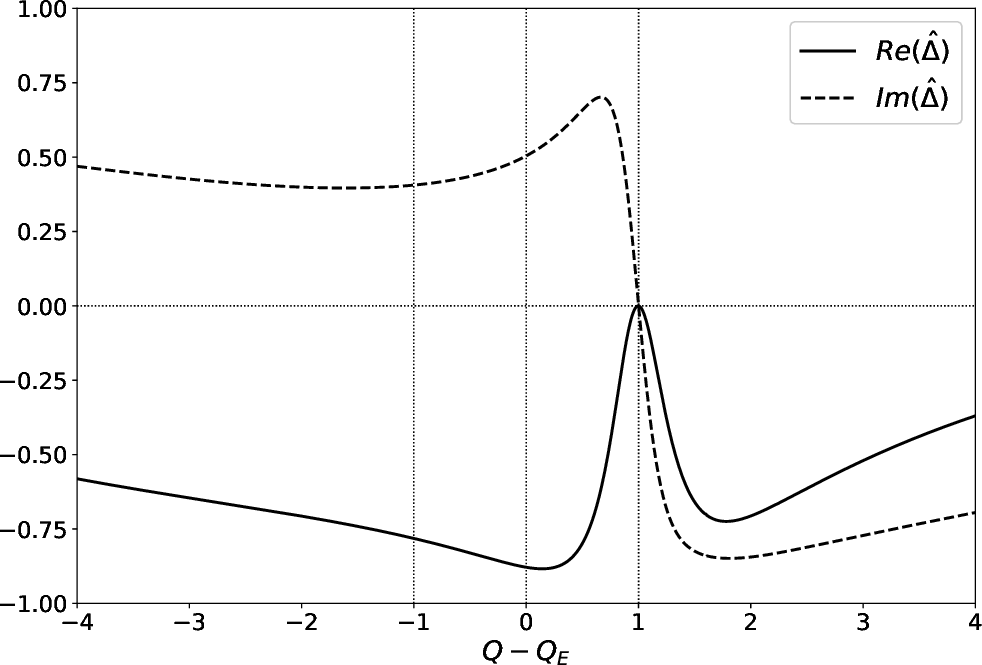Let us make use of the so-called Riccati transformation [5,18],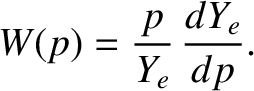(5.120)

Equation (5.78) yields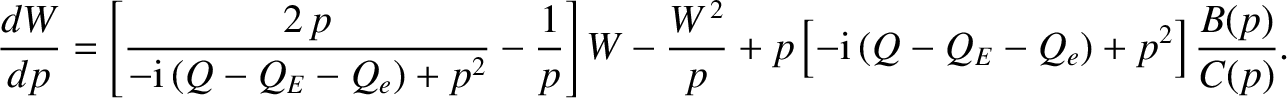(5.121)

According to Equation (5.115), the small-behavior of the solution to the previous equation is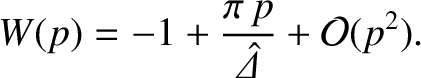(5.122)

Likewise, according to Equation (5.119), the large-behavior of the solution is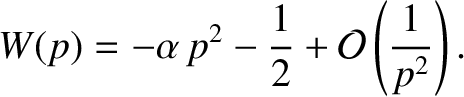(5.123)

Equation (5.121) is conveniently solved numerically by launching a solution of the form (5.123) at large, and then integrating backward to small. Equation (5.122) yields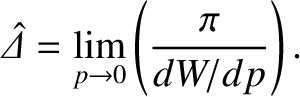(5.124)

Figure 5.5 shows a numerical solution of the resonant layer equation for a low-field tokamak fusion reactor. This calculation is made with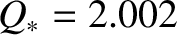,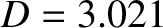,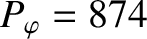,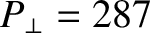, and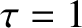, assuming that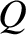is real. (See Table 5.1.) Note that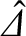parameterizes the amplitude and phase of a shielding current that is driven inductively at the rational surface, in response to a rotating tearing perturbation in the outer region, and acts to suppress magnetic reconnection at the surface . It can be seen that the shielding current is zero when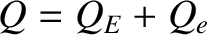, which is equivalent to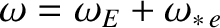. In other words, the shielding current is zero when the tearing perturbation in the outer region rotates at the frequency of a naturally unstable tearing mode at the rational surface [2,11]. (See Chapter 6.) The shielding current clearly increases linearly with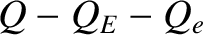when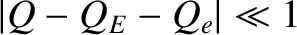, but saturates in magnitude as.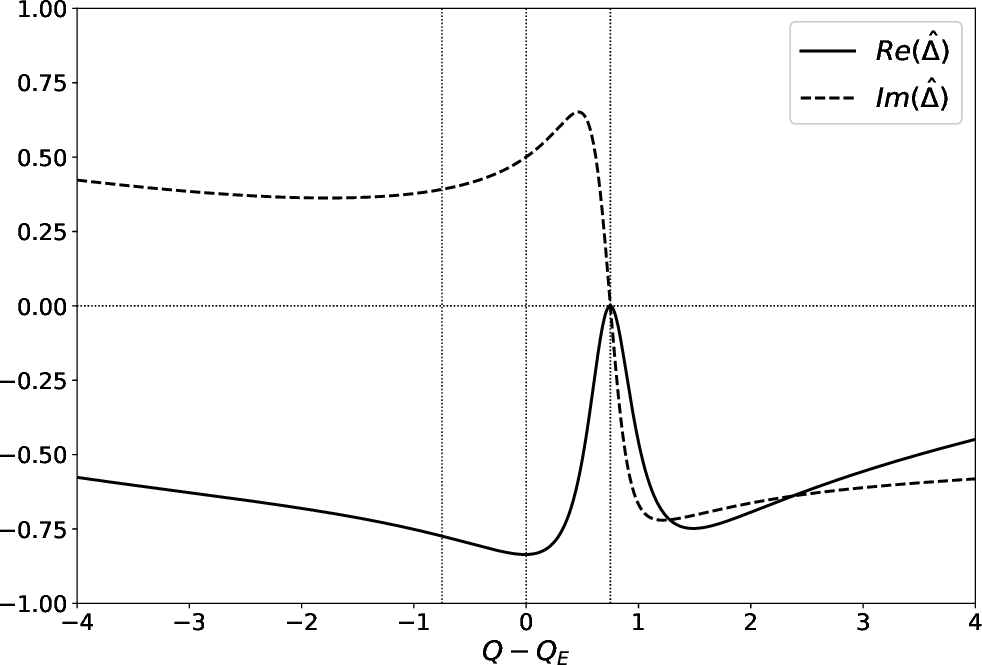Figure 5.6 shows a numerical solution of the resonant layer equation for a high-field tokamak fusion reactor. This calculation is made with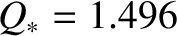,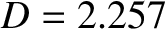,,, and, assuming thatis real. (See Table 5.1.) Note that the figure is very similar to Figure 5.5, indicating that the resonant layer responses in low-field and high-field tokamak fusion reactors do not differ substantially from one another.